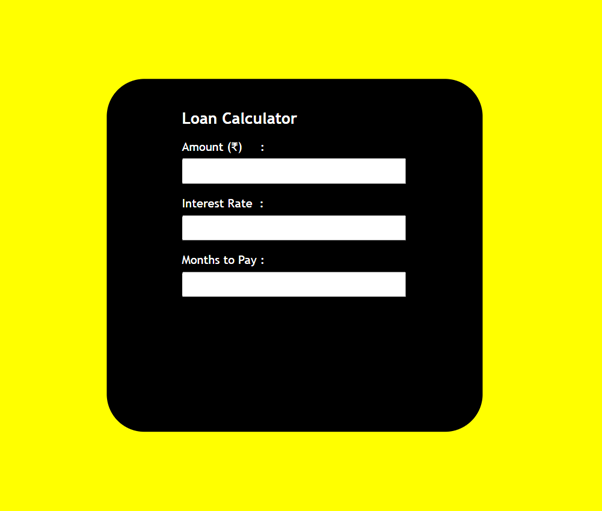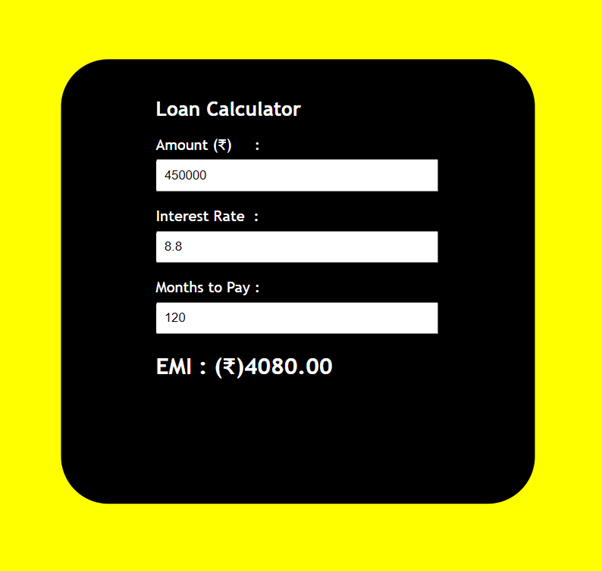# Design a Loan Calculator using JavaScript

• Difficulty Level : Medium
• Last Updated : 23 Feb, 2021

The Loan Calculator can be used to calculate monthly EMI of the loan by taking the total amount, months to repay and the rate of interest.

Approach: The approach is very simple, we will take 3 inputs from user i.e. amount (total loan amount), rate (the rate of interest) and months (number o months to repay). Using these three, we can calculate the total amount. At last we will display the total amount.

Hey geek! The constant emerging technologies in the world of web development always keeps the excitement for this subject through the roof. But before you tackle the big projects, we suggest you start by learning the basics. Kickstart your web development journey by learning JS concepts with our JavaScript Course. Now at it's lowest price ever!

Formula:

```interest = (amount * (rate * 0.01))/months;
total = ((amount/months) + interest);```

Using HTML we are designing the simple structure and giving style using CSS(internal CSS). At the time of input we are calling calculate() function and displaying the result. The calculate() function takes input using HTML attribute named – onchange (the onchange attribute fires the moment when the value of the element is changed).

Prerequisite: Basics concepts of HTML, CSS and JavaScript.

Implementation: We will make two separate files i.e. HTML and JavaScript and link the JavaScript file within HTML file.

• HTML – (index.html)
• JavaScript – (app.js)

HTML File:

## index.html

 ```<``html` `lang``=``"en"``>`` ` `<``head``>``    ``<``title``>Loan Calculator`` ` `    ``<``style``>``        ``body {``            ``background-color: yellow;``            ``font-family: 'Trebuchet MS';``        ``}``         ` `        ``h1 {``            ``font-size: 35px;``        ``}``         ` `        ``h1 {``            ``font-size: 21px;``            ``margin-top: 20px;``        ``}``         ` `        ``.calculator {``            ``width: 400px;``            ``height: 450px;``            ``background-color: black;``            ``position: absolute;``            ``left: 50%;``            ``top: 50%;``            ``transform: translateX(-50%) translateY(-50%);``            ``padding: 20px 0px 0px 100px;``            ``border-radius: 50px;``            ``color: white;``        ``}``         ` `        ``input {``            ``padding: 7px;``            ``width: 70%;``            ``margin-top: 7px;``        ``}``    `````` ` `<``body``>``    ``<``div` `class``=``"calculator"``>``        ``<``h1``>Loan Calculator`` ` `        ````        ``<``p``>Amount (₹)     :``            ``<``input` `id``=``"amount"` `type``=``"number"` `            ``onchange``=``"Calculate()"``>``        ```` ` `        ``<``p``>Interest Rate  :``            ``<``input` `id``=``"rate"` `type``=``"number"` `            ``onchange``=``"Calculate()"``>``        ```` ` `        ``<``p``>Months to Pay :``            ``<``input` `id``=``"months"` `type``=``"number"` `            ``onchange``=``"Calculate()"``>``        ```` ` `        ``<``h2` `id``=``"total"``>``    ```` ` `    ``<``script` `src``=``"app.js"``>```` ` ``

JavaScript File:

## app.js

 `function` `Calculate() {`` ` `    ``// Extracting value in the amount ``    ``// section in the variable``    ``const amount = document.querySelector(``"#amount"``).value;`` ` `    ``// Extracting value in the interest``    ``// rate section in the variable``    ``const rate = document.querySelector(``"#rate"``).value;`` ` `    ``// Extracting value in the months ``    ``// section in the variable``    ``const months = document.querySelector(``"#months"``).value;`` ` `    ``// Calculating interest per month``    ``const interest = (amount * (rate * 0.01)) / months;``     ` `    ``// Calculating total payment``    ``const total = ((amount / months) + interest).toFixed(2);`` ` `    ``document.querySelector(``"#total"``)``        ``.innerHTML = ``"EMI : (₹)"` `+ total;``}  `

Outputs:My Personal Notes arrow_drop_up